# Find the squares of the following numbers:`
Question:

Find the squares of the following numbers:

(i) 127

(ii) 503

(iii) 451

(iv) 862

(v) 265

Solution:

We will use visual method as it is the most efficient method to solve this problem.

(i) We have:

127 = 120 + 7

Hence, let us draw a square having side 127 units. Let us split it into 120 units and 7 units.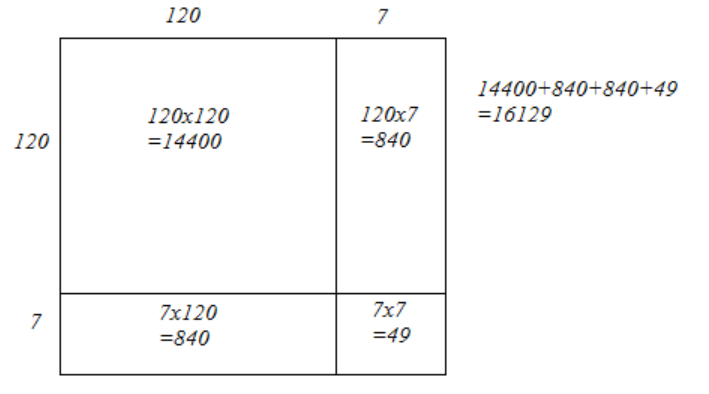Hence, the square of 127 is 16129.

(ii) We have:

503 = 500 + 3

Hence, let us draw a square having side 503 units. Let us split it into 500 units and 3 units.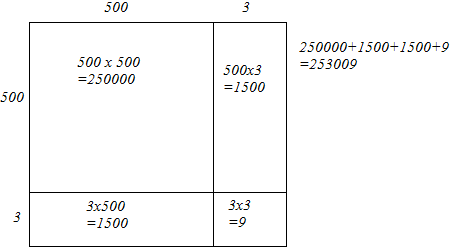Hence, the square of 503 is 253009.

(iii) We have:

451 = 450 + 1

Hence, let us draw a square having side 451 units. Let us split it into 450 units and 1 units.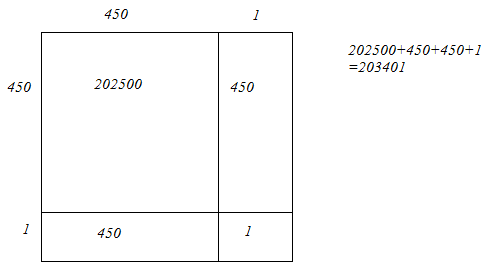Hence, the square of 451 is 203401.

(iv) We have:

862 = 860 + 2

Hence, let us draw a square having side 862 units. Let us split it into 860 units and 2 units.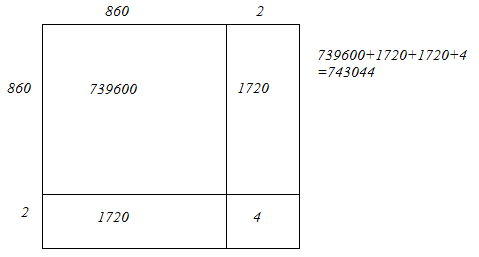Hence, the square of 862 is 743044.

(v) We have:

265 = 260 + 5

Hence, let us draw a square having side 265 units. Let us split it into 260 units and 5 units.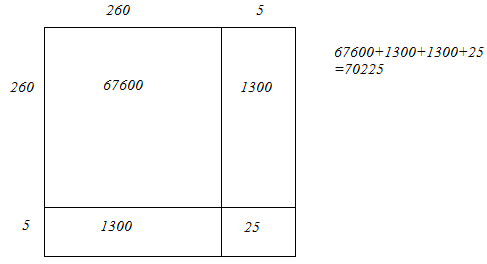Hence, the square of 265 is 70225.